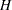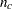Computational Resources

Due to the complex nature of survey data analysis, the SURVEYREG  procedure requires more memory than an analysis of the same regression model by the GLM procedure. For details about the amount of memory related to the modeling, see the section Computational Resources in Chapter 41, The GLM Procedure.

The memory needed by the SURVEYREG  procedure to handle the survey design is described as follows.

Let

•be the total number of strata

•be the total number of clusters in your sample across allstrata, if you specify a CLUSTER statement

•be the total number of parameters in the model

The memory needed (in bytes) isFor a cluster sample, the additional memory needed (in bytes) isThe SURVEYREG procedure also uses other small amounts of additional memory. However, when you have a large number of clusters or strata, or a large number of parameters in your model, the memory described previously dominates the total memory required by the procedure.